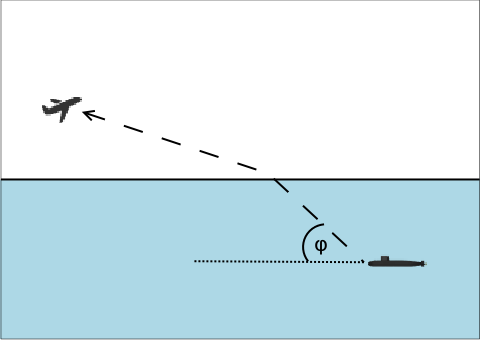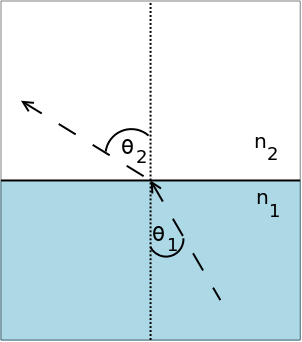시간 제한메모리 제한제출정답맞힌 사람정답 비율
1 초 256 MB26151557.692%

## 문제

A submarine is using a communications laser to send a message to a jet cruising overhead. The sea surface is flat. The submarine is cruising at a depth d below the surface. The jet is at height h above the sea surface, and a horizontal distance x from the sub. The submarine turns toward the jet before starting communications, but needs to know the angle of elevation, φ, at which to aim the laser.When the laser passes from the sea into the air, it is refracted (its path is bent). The refraction is described by Snell's law, which says that light approaching the horizontal surface at an angle θ1, measured from the vertical, will leave at an angle θ2, given by the formula

sin θ1 / sin θ2 = n1 / n2

where n1 and n2 are the respective refraction indices of the water and air. (The refraction index of a material is inversely proportional to how fast light can travel through that material.)## 입력

Each test case consists of a single line of input containing 5 space-separated floating point numbers:

• d, the depth of the submarine (specifically, of the laser emitter) in feet, 1 <= d <= 800
• h, the height of the plane in feet, 100 <= h <= 10,000
• x, the horizontal distance from the sub to the plane in feet, 0 <= x <= 10,000
• n1, the refractive index of water, 1.0 < n1 <= 2.5
• n2, the refractive index of air, 1.0 <= n2 < n1

Input ends with a line containing 5 zeroes (0 0 0 0 0).

## 출력

For each test case, print a single line containing the angle of elevation φ at which the submarine should aim its laser to illuminate the jet.

The angle should be displayed in degrees and rounded to the closest 1/100 of a degree. Exactly two digits after the decimal point should be displayed.

## 예제 입력 1

600 600 1000 1.333 1.01
600 1200 4000 1.5 1.01
400 100 10000 2.5 1.01
0 0 0 0 0


## 예제 출력 1

44.37
11.51
2.30